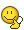BrainDen.com - Brain Teasers

## Question

You want to set your computer's clock to be synchronized with the clock of a distant server.

You communicate with the server by sending packets (messages) the time it takes for a packet to travel from your computer to the server is t1 which is consistent(but unknown) and the time it takes from the server to your computer is t2 also consistent and unknown, t1 isn't necessarily equal to t2.

Assuming you can write any code you want on your computer and the server, can you think of a way to synchronize your clocks?

Edited by Anza Power

## Recommended Posts

• 0

I'm tired so my reasoning is probably flawed:

Send a message to get any instant response, the time this is recieved will give you t1+t2.

Send a message requesting the time, this will give the time as (actualy time)-t2

Send a message requesting the error in the recieved time, say this message is sent at actual time=T, so the time sent will be T-t2, this will be recieved as T+t1-t2 and at actual time T+t1 so the server can calculate the error as t2 (since the server has all information required).

The server can then send t2 as a message and this can be used to calculate t1 and the actual time can easily be worked out from there.

##### Share on other sites
• 0

I'm tired so my reasoning is probably flawed:

Send a message to get any instant response, the time this is recieved will give you t1+t2.

Send a message requesting the time, this will give the time as (actualy time)-t2

Send a message requesting the error in the recieved time, say this message is sent at actual time=T, so the time sent will be T-t2, this will be recieved as T+t1-t2 and at actual time T+t1 so the server can calculate the error as t2 (since the server has all information required).

The server can then send t2 as a message and this can be used to calculate t1 and the actual time can easily be worked out from there.

In the third step, wouldn't the time received by the server still be T-t2?

##### Share on other sites
• 0

Ask the server for time. Let us say you sent the request at time T; this request reaches the server at time T+t1. The server sends the time T+t1.
So, you get the time T+t1, but the actual time now is T+t1+t2 when you get the time.

Program such that you send another request at the very instant you get the first response from the server.

So, at time T+t1+t2, you send the second request, and the time you receive from the server is T+2t1+t2 while the actual time is T+2t1+2t2 when you get this time.

Calculate the difference between the two times recieved. You now know how much is t1+t2.

Now, ask the server to send to send you the time (Tn) exactly n seconds later; for example you ask the server what time will it be after 30 seconds.

As before, you will get the time Tn+t1, when the actual time is Tn+t1+t2.

Subtract t1+t2 from the time Tn+t1 received from the server and set your computer clock to Tn after n seconds.

##### Share on other sites
• 0

Ask the server for time. Let us say you sent the request at time T; this request reaches the server at time T+t1. The server sends the time T+t1.

So, you get the time T+t1, but the actual time now is T+t1+t2 when you get the time.

Program such that you send another request at the very instant you get the first response from the server.

So, at time T+t1+t2, you send the second request, and the time you receive from the server is T+2t1+t2 while the actual time is T+2t1+2t2 when you get this time.

Calculate the difference between the two times recieved. You now know how much is t1+t2.

Now, ask the server to send to send you the time (Tn) exactly n seconds later; for example you ask the server what time will it be after 30 seconds.

As before, you will get the time Tn+t1, when the actual time is Tn+t1+t2.

Subtract t1+t2 from the time Tn+t1 received from the server and set your computer clock to Tn after n seconds.

if you subtract that then you'd have Tn-t2

##### Share on other sites
• 0

Ok now here's what I think:

I think this is impossible, but can't get my head around a good proof.

You can simplify the problem by asking just if you can distinguish between two cases, let's say the RTT (round trip time) which is t1+t2 is exactly 1 second, and it is the case that {t1=1, t2=0} or {t1=0, t2=1} let's name them situations A and B respectively, imagine you are the server and you are the one who sends the first message at time T=0, in both A and B the things you send and receive are exactly the same, on the client sid the arrival times differ but they are all shifted by exactly 1 second and since client has no fixed point in time to compare stuff to he cannot distinguish the cases either

##### Share on other sites
• 0

I take that back, it probably is impossible. The three equations with three unknowns I could set up turned out to be degenerate.## Join the conversation

You can post now and register later. If you have an account, sign in now to post with your account.×   Pasted as rich text.   Paste as plain text instead

Only 75 emoji are allowed.

×   Your previous content has been restored.   Clear editor

×   You cannot paste images directly. Upload or insert images from URL.